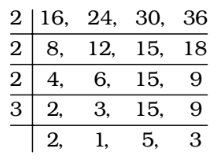Home » Aptitude » LCM and HCF » Question

#### LCM and HCF

1. The largest number of five digits which, when divided by 16, 24, 30, or 36 leaves the same remainder 10 in each case, is :
1. 99279
2. 99370
3. 99269
4. 99350
##### Correct Option: B

The n digit largest number which when divided by p, q, r leaves remainder ‘a’ will be
Required number = [n – digit largest number – R] + a
where, R is the remainder obtained when n–digit largest number is divided by the L.C.M of p, q, r.
We will find the LCM of 16, 24, 30 and 36.∴ LCM = 2 × 2 × 2 × 3 × 2 × 5 × 3 = 720
The largest number of five digits = 99999
On dividing 99999 by 720, the remainder = 639
∴ The largest five-digit number divisible by 720
= 99999 – 639 = 99360
∴ Required number = 99360 + 10 = 99370